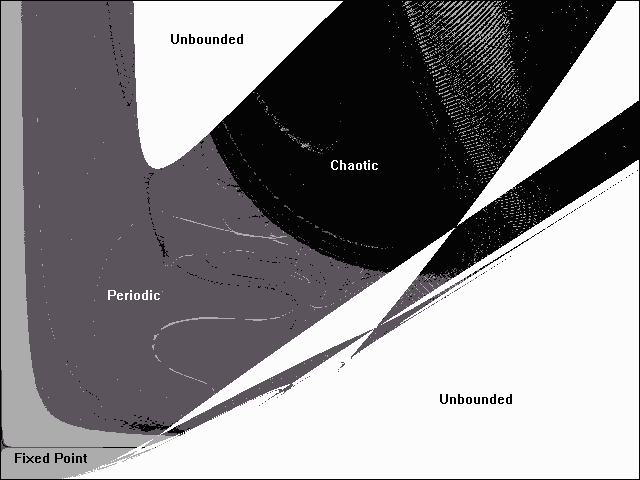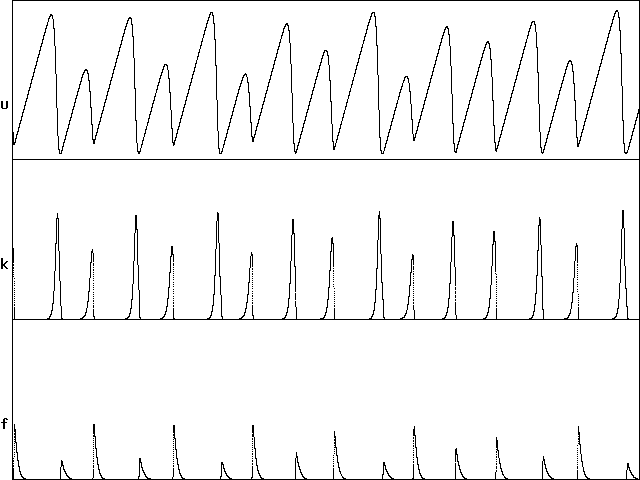# Chaos in Edge Localized Modes

J. C. Sprott
Department of Physics, University of Wisconsin, Madison, WI 53706, USA
March 26, 1999
Revised October 16, 2003

Fusion plasmas magnetically confined by tokamak devices often exhibit relaxation oscillations in hydrogen-alpha (or deuterium-alpha) emission and other quantities in the outer edge of the plasma when in the high-confinement mode (H-mode) at sufficiently high plasma pressure (beta).  These oscillations are called edge localized modes (ELMs).  Although the ELMs degrade the confinement, they are potentially beneficial for removing helium ash from a fusion plasma.  The ELMs are approximately periodic, but the period and amplitude generally exhibit variations which are presumably chaotic.  Many theoretical models for the H-mode and ELMs have been proposed.  A particularly simple model was recently advanced by  H. Sugama and W. Horton in Plasma Phys. Control. Fusion 37, 345-362 (1995).  This note shows that their model leads to chaotic dynamics with some choices of parameters, and it characterizes the chaos thus produced.

The Sugama-Horton model describes the temporal evolution of three variables, the potential energy u associated with the pressure profile, the turbulent kinetic energy k, and the kinetic energy f associated with the background shear flow.  In normalized form, these variables evolve according to the equations:

du/dt = q - u1/2k

dk/dt = u1/2k - cu-1/2fk - d-1(u)k2

df/dt = cu-1/2fk - cm(u)f

where q is the normalized potential energy input, which is a controllable parameter, and c is a nondimensional numerical constant.  The function d(u) is the L-mode anomalous diffusivity, which depends on u through its dependence on background temperature.  The function m(u) is related to the ion collisional viscosity.  Following Sugama and Horton, we take d(u) = u and m(u) = u-3/2(0.95 + 0.05u5/2).

Sugama and Horton found limit-cycle solutions for q = 1.8 and c = 5.0 but did not observe chaos.  Here we explore the dynamical behavior of the above system over the whole space of 0 < q < 15 and 0 < c < 20.  The equations were solved using a fourth-order Runge-Kutta integrator for 32000 steps with a step size of 0.02 and initial conditions of u(0) = k(0) = f(0) = 0.05.  The character of the solution was identified by calculating the largest Lyapunov exponent.  Exponents greater than 0.008 were assumed to be chaotic, exponents less than -0.005 were assumed to be fixed points, and other exponents were assumed to be limit cycles (although they could also be toruses).  A solution was assumed to be unbounded if |u| ever exceeds 5000.  The calculation was implemented in PowerBASIC using the source and (DOS) object code provided.  The resulting plot is shown below:c

It is likely that some of the unbounded solutions above are a result of initial conditions outside the basin of attraction.  It would be even more computationally intensive to test for this possibility.

With a slight modification of the code, the Lyapunov exponent in the chaotic region can be displayed as a gray scale on the same axes as above.  The source and object code for accomplishing this are provided.  The gray scale is linear in the range of Lyapunov exponents from 0 to 0.2 in the plot shown below:

qc

The smallest parameter values that put the dynamics comfortably into the chaotic region are q = 9 and c = 9.  The remainder of this note will concentrate on that case.  The time evolution of the three variables were calculated over 32000 time steps starting from a point on the attractor with a step size of 0.002 using the source and object code provided, and the chaotic results are shown below:time

In a much longer run, the Lyapunov exponents (base-e) converge to about (0.085, 0, -0.938), giving a Kaplan-York dimension of about 2.090.  An animated view of the attractor is shown below:In the image above, the k axis points upward, and the object rotates in the u-f plane.